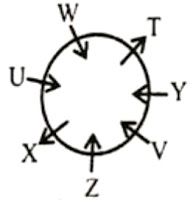Reasoning Quiz for NIACL, India Post

Directions Q. 1-5): Study the following information carefully and answer the questions given below:Twelve persons N, P, Q, R, S, T, U, V, W, X, Y and Z are sitting in two parallel rows. Row 1 is facing west and row 2 is facing east. P, Q, R, S, T and U are facing west. Each person of row 1 is facing the other person of row 2.
V sits at one of the ends of a row and second to the left of Y. There are two persons between Z and N. There is only one person between T and S. U sits third to the right of Q and is not opposite either V or Y. S and T are not opposite V. P and S are adjacent to each other. N is not adjacent to W, who is not opposite T.

1). Who among the following is opposite Q?
a) Y
b) Z
c) W
d) N
e) None of these

2). How many persons are there between S and R?
a) One
b) Two
c) Three
d) None
e) None of these

3). Which of the following statements is/are true about P?
a) S does not sit adjacent to P.
b) Z sits opposite the person who sits third to the left of P.
c) Y sits opposite P.
d) N does not sit opposite the person who sits on the immediate right of P.
e) None of these

4). Who among the following sit at the extreme ends of row 2?
a) R,V
b) V, X
c) T, U
d) N, Z
e) None of these

5). Who among the following sits second to the right of N?
a) W
b) V
c) Z
d) X
e) None of these

Directions (Q. 6-11): Study the following information carefully and answer the questions given below:

Seven persons T, U, V, W, X, Y and Z are sitting around a circular table. Two of them are not facing the centre and the rest are facing the centre. V is sitting third to the left of W and both are facing the centre. X is not an immediate neighbour of W or V. The one who is exactly between W and Y is not facing the centre. Z is sitting third to the right of T and is facing the centre. One of the immediate neighbours of U is not facing the centre.

6). Who among the following are not facing the centre?

a) U and X
b) Y and V
c) T and X
d) Can't be determined
e) None of these

7). Who among the following is second to the left of X?

a) V
b) W
c) T
d) Y
e) None of these

8). Who among the following is third to the left of V?
a) Y
b) U
c) T
d) Can't be determined
e) None of these

9). What is the position of Y with respect to U?
a) Third to the right
b) Second to the left
c) Third to the left
d) Fifth to the right
e) None of these

10). Which of the following statements is true?

a) Y sits on the immediate left of T.
b) The person who is exactly between Y and Z is facing the centre.
c) The person who is exactly between U and Z is not facing the centre.
d) Only 2) and 3) are true
e) None is true

11). Who among the following are immediate neighbours of X?
a) UZ
b) WY
c) TZ
d) W
e) None of these

1)b
2)b
3)c
4)b
5)a6).c)
7).a)
8).b)
9).c)
10).d)
11).a)